# 圆锥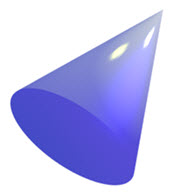• 平的底面
• 一个曲面
• 不是多面体，因为有个曲面• 圆锥尖端的点叫顶点
• 平的部分叫底面

## 圆锥是旋转的三角形## 圆锥的表面积

• 底面的面积 = π × r2
• 侧面积 = π × r × s
• 也可以写成：侧面积 = π × r × √(r2+h2)

### 例子：h = 7，r = 2

 底面的面积 = π × r2 = π × 22 = 4π ≈ 12.57
 侧面积 = π × r × √(r2+h2) = π × 2 × √(22+72) = π × 2 × √(4+49) = 2√(53) × π ≈ 45.74

## 圆锥的体积

### 例子：h = 7，r = 2

 体积 = 1 3 π × r2 × h = 1 3 π × 22 × 7 = 28 3 π ≈ 29.32

## 圆锥与圆柱的体积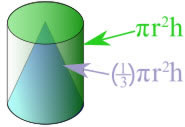圆柱的体积是： π × r2 × h 圆锥的体积是： 1 3 π × r2 × h

## 不同形状的圆锥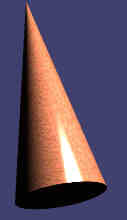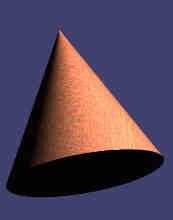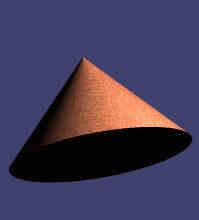## 施工锥形筒